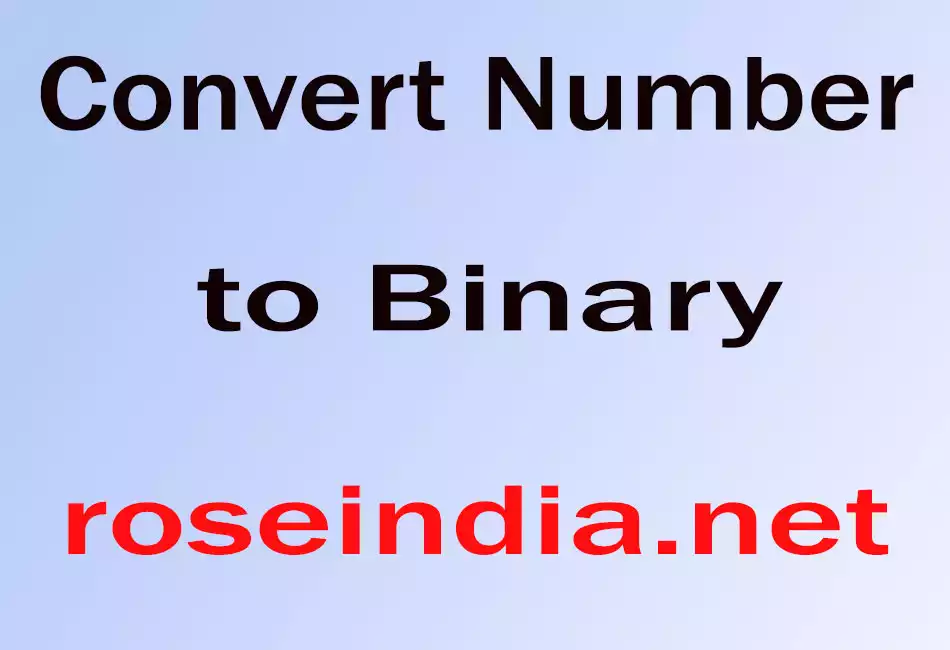# Convert Number to Binary

In this section, you will learn to convert a number to a binary (0,1).### Convert Number to Binary

In this section, you will learn to convert a number to a binary (0,1).# Convert Number to Binary

In this section, you will learn to convert a number to a binary (0,1). The given number is a decimal format and the following program to calculate its binary.

Code Description:

This program takes a decimal number at the console. This number is as a string format so, it must be converted into an integer type data using the Integer.parseInt() method. The toBinaryString() method is used for converting an integer type data into a binary.

Here is the code of this program:

 ``` import java.io.*; import java.lang.*; public class NumberToBinary {   public static void main(String[] args) throws IOException{   BufferedReader bf= new BufferedReader(new InputStreamReader(System.in));   System.out.println("Enter any number:");   String sn = bf.readLine();   int i = Integer.parseInt(sn);   String s = Integer.toBinaryString(i);   System.out.println("Binary number is:" + s);   } }```

`Download of this program:`

`Output of this program given below.`

 C:\corejava>java NumberToBinary Enter any number: 25 Binary number is:11001 C:\corejava> _